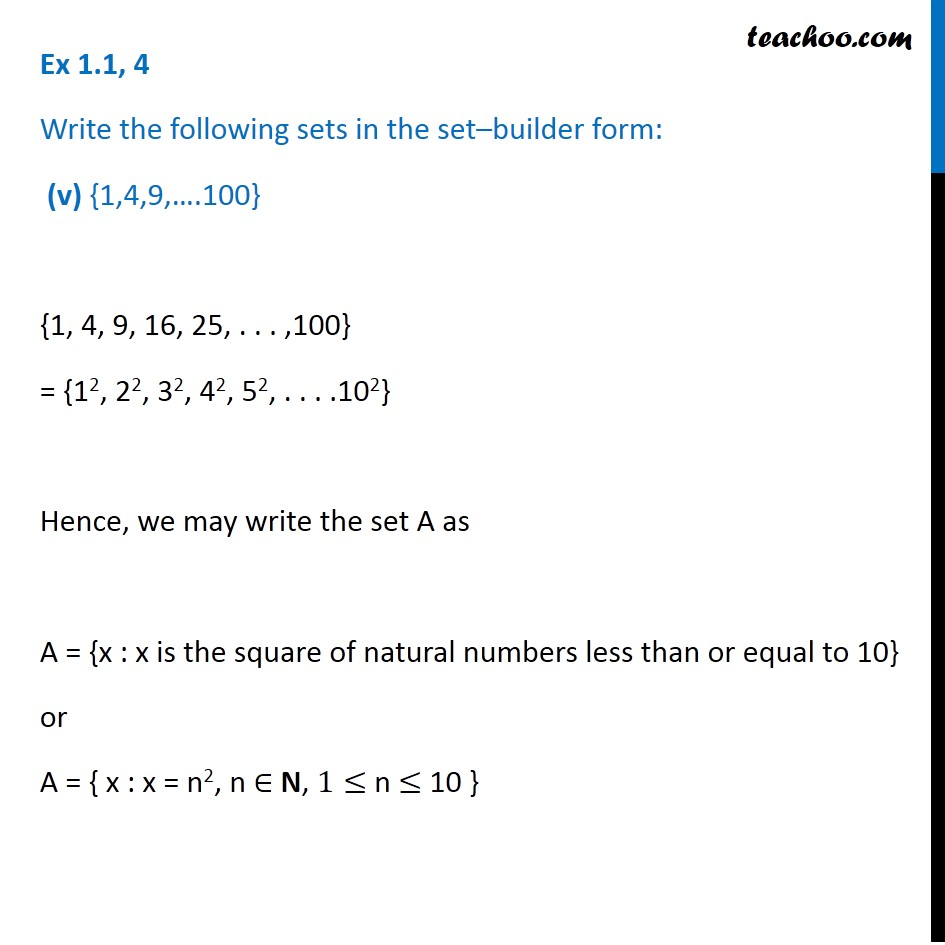1. Chapter 1 Class 11 Sets (Term 1)
2. Serial order wise
3. Ex 1.1

Transcript

Ex 1.1, 4 Write the following sets in the set–builder form: (v) {1,4,9,….100} {1, 4, 9, 16, 25, . . . ,100} = {12, 22, 32, 42, 52, . . . .102} Hence, we may write the set A as A = {x : x is the square of natural numbers less than or equal to 10} or A = { x : x = n2, n ∈ N, 1≤ n ≤ 10 }